# Unflatten Pixmap VI

## LabVIEW 2018 Help

Edition Date: March 2018
Part Number: 371361R-01
View Product InfoLabVIEW 2016 HelpLabVIEW 2017 HelpLabVIEW 2018 HelpLabVIEW 2019 HelpLabVIEW 2020 Help

Owning Palette: Graphics Formats VIs

Requires: Base Development System

Converts a cluster of image data into a 2D array.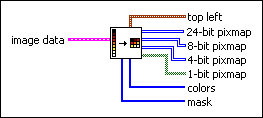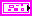image data describes the image you want to draw or manipulate. If you wire 32-bit image data to this VI, the VI returns a 24-bit pixmap.image type is reserved for future use.image depth specifies the color depth of the image, which is the number of bits to use to describe the color of each pixel in the image. Valid values include 1, 4, 8, and 24 bits per pixel. image depth affects how LabVIEW interprets the values of image and colors.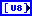image is an array of bytes that describes the color of each pixel in the image in raster order. The value of image depth determines how LabVIEW interprets the value of this input. If image depth is 24, each pixel has three bytes to describe its color. The first byte for each pixel describes the red value, the second byte describes the green value, and the third byte describes the blue value.

If image depth is 8, each pixel has one byte to describe its color. The value of each byte corresponds to an element in colors, which stores 32-bit RGB values where the most-significant byte is zero, followed in order by red, green, and blue values. Valid values include 0 through 255.

If image depth is 4, the behavior is similar to when image depth is 8 except valid values in image include 0 through 15.

If image depth is 1, any value of zero in image corresponds to element 0 in colors. All other values correspond to element 1 in colors.mask is an array of bytes in which each bit describes mask information for a pixel. The first byte describes the first eight pixels, the second byte describes the next eight pixels, and so on. If a bit is zero, LabVIEW draws the corresponding pixel as transparent. If the array is empty, LabVIEW draws all pixels without transparency. If the array does not contain a bit for each pixel in the image, LabVIEW draws any pixels missing from the array without transparency.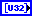colors is an array of RGB color values that correspond to the values in image. The value of image depth determines how LabVIEW interprets the value of this input. colors stores 32-bit RGB values where the most-significant byte is zero, followed in order by red, green, and blue values. Valid values include 0 through 255.

If image depth is 24, LabVIEW ignores this input.

If image depth is 8, the array can have 256 elements.

If image depth is 4, the array can have 16 elements.

If image depth is 1, the array can have 2 elements.Rectangle is a cluster that contains coordinates that describe the bounding rectangle of the image. The bottom and right edges of the rectangle bounds do not contain image pixels. Horizontal coordinates increase to the right, and vertical coordinates increase to the bottom.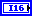left is the horizontal coordinate of the left edge of the rectangle.top is the vertical coordinate of the top edge of the rectangle.right is the horizontal coordinate of the right edge of the rectangle.bottom is the vertical coordinate of the bottom edge of the rectangle.top left is a cluster that contains a horizontal (x) and a vertical (y) component.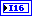x is the horizontal coordinate that increases to the right.y is the vertical coordinate that increases to the bottom.24-bit pixmap returns the 2D array of data to draw as a pixmap. The dimensions of the pixmap match the dimensions of this array.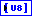8-bit pixmap returns the 2D array of data to draw as a pixmap. The dimensions of the pixmap match the dimensions of this array. The VI uses the data as indexes in the colors output.4-bit pixmap returns the 2D array of data to draw as a pixmap. The dimensions of the pixmap match the dimensions of this array. The VI uses the data as indexes in the colors output.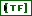1-bit pixmap returns the 2D array of data to draw as a bitmap. The dimensions of the bitmap match the dimensions of this array. Elements that are FALSE map to element 0 in the colors output and elements that are TRUE map to element 1.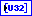colors is an array of RGB color values that correspond to the values in the pixmap output. For the 24-bit pixmap, LabVIEW ignores this output. For the 8-bit pixmap, the array can have 256 elements. For the 4-bit pixmap, the array can have 16 elements. For the 1-bit pixmap, the array can have 2 elements.mask is an array of bytes in which each bit describes mask information for a pixel. The first byte describes the first eight pixels, the second byte describes the next eight pixels, and so on. If a bit is zero, LabVIEW draws the corresponding pixel as transparent. If the array is empty, LabVIEW draws all pixels without transparency. If the array does not contain a bit for each pixel in the image, LabVIEW draws any pixels missing from the array without transparency.

## Unflatten Pixmap Details

When you use a Graphics Formats VI to read a graphics file, the VI returns the image data in a cluster. Use the Draw Unflattened Pixmap VI to display the data directly or use the Unflatten Pixmap VI to convert the data to a more useful 2D representation.

In this VI, only one of the various pixmap outputs (24-bit, 8-bit, 4-bit, or 1-bit) is valid at a time. In other words, this VI will not produce an output of two different pixmap values.